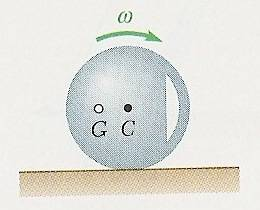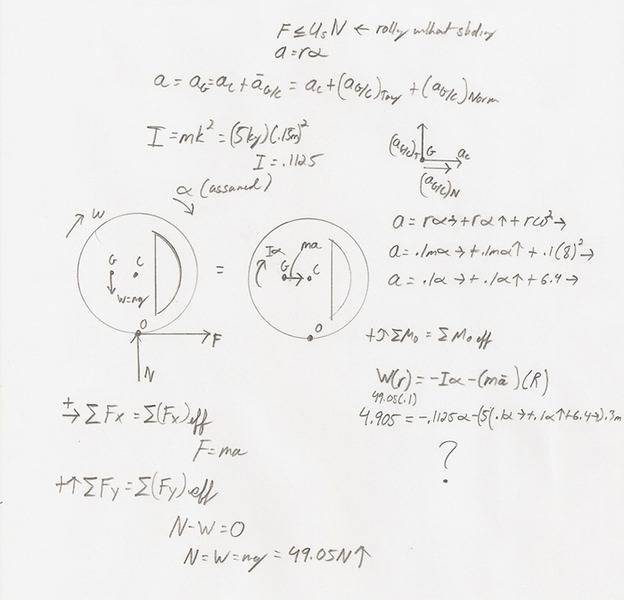# Rolling motion of an unbalanced disk

## Homework Statement

The mass center G of a 5kg wheel of radius R = 300 mm is located at a distance r = 100 mm from its geometric center C. The centroidal radius of gyration is k = 150 mm. As the wheel rolls without sliding, its angular velocity varies and it is observed that it is = 8 rad/s in position shown. Determine the corresponding angular acceleration of the wheel.## Homework Equations

Ac=Ag=Ac + Ag/c = Ac + (Ag/c)tangent + (Ag/c) Normal
I= mk^2
a=r$$\alpha$$

## The Attempt at a Solution